Filters
Size

# Sale Tees

Filters

Selected Filters 

Sold Out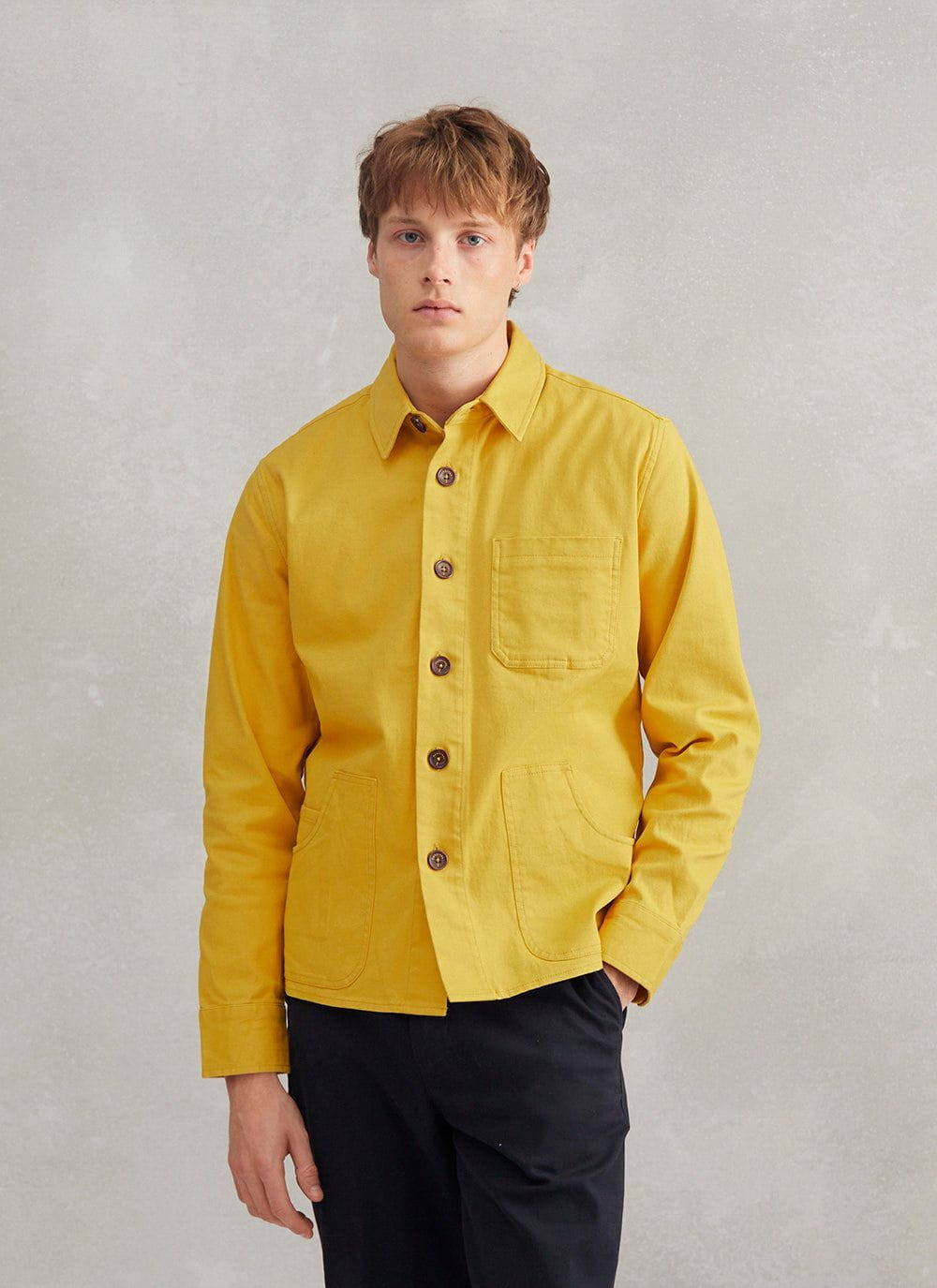• XS
• S
• M
• L
• XL
• XXL
• Select Size• XS
• S
• M
• L
• XL
• XXL
• Select Size• XS
• S
• M
• L
• XL
• XXL
• Select Size
Running Low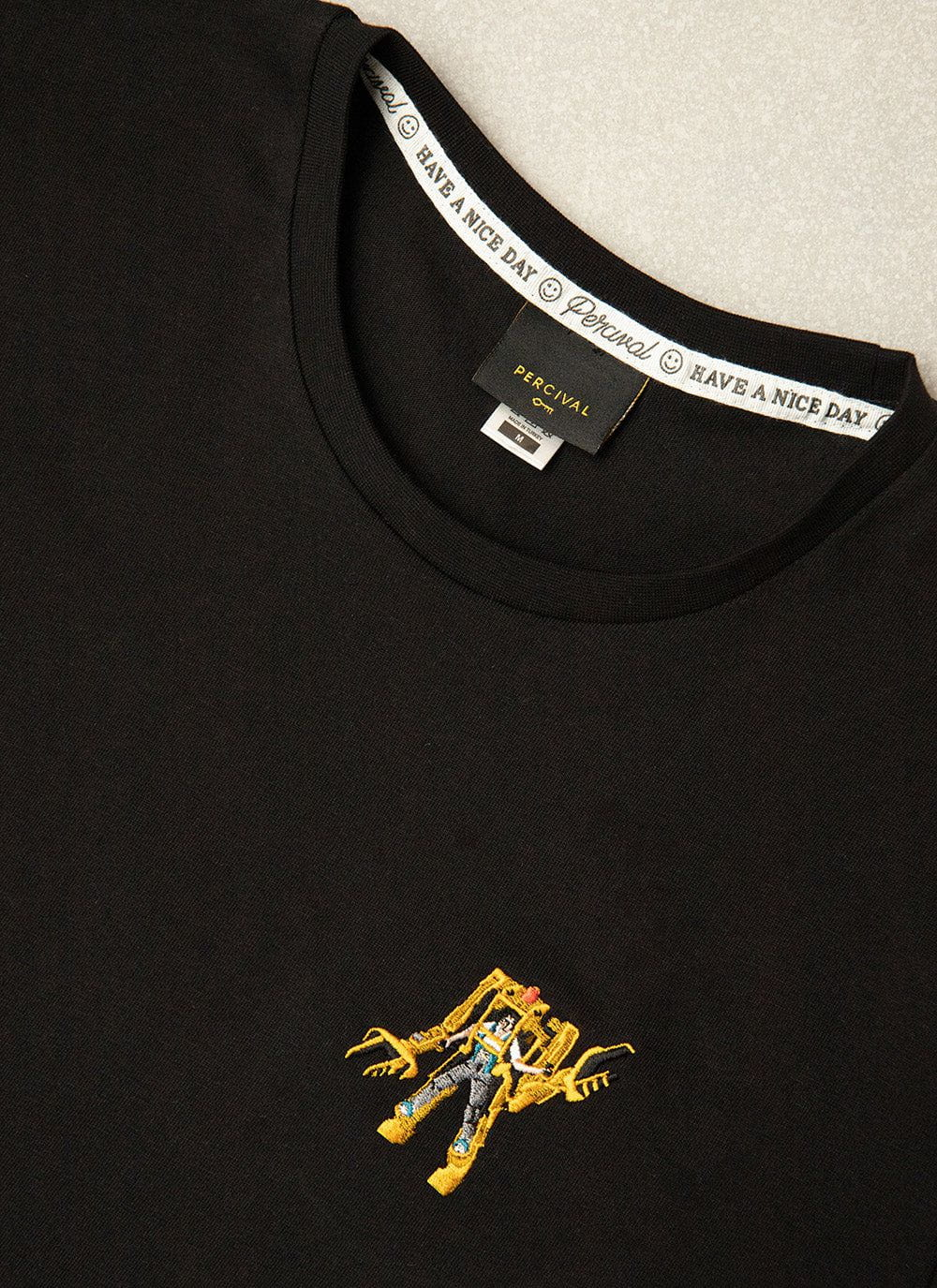• XS
• S
• M
• L
• XL
• XXL
• Select Size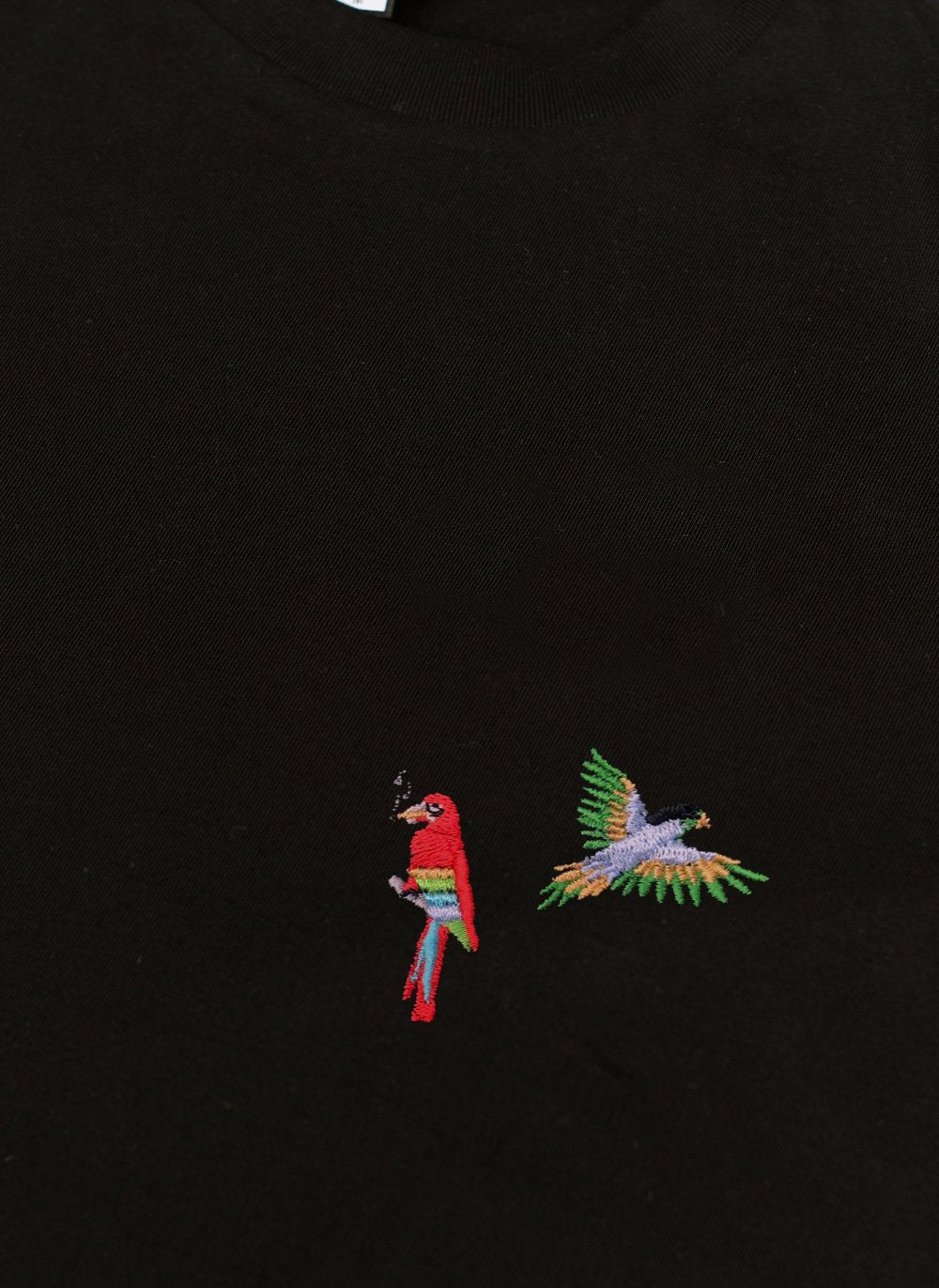• XS
• S
• M
• L
• XL
• XXL
• Select Size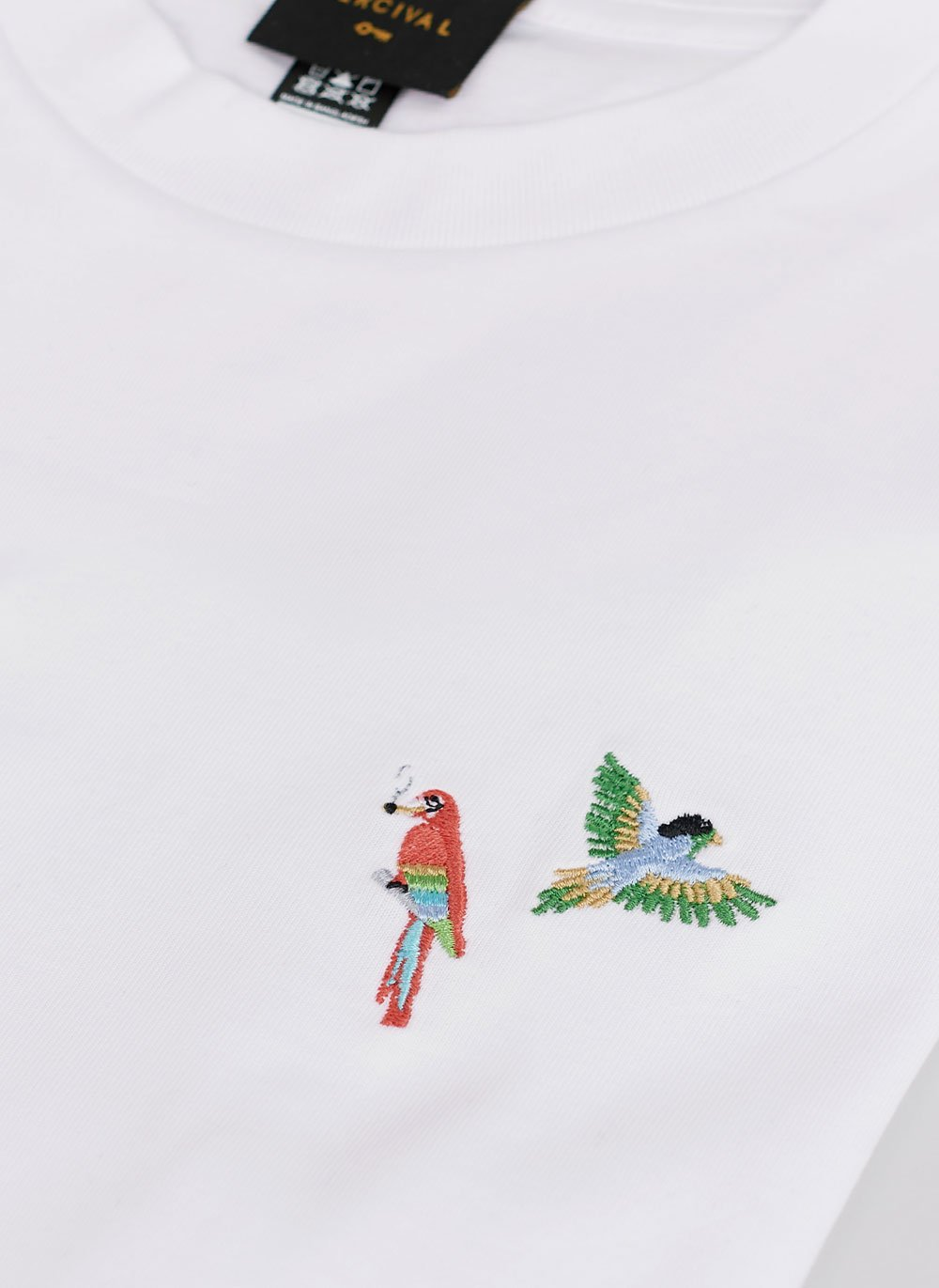• XS
• S
• M
• L
• XL
• XXL
• Select Size• XS
• S
• M
• L
• XL
• XXL
• Select Size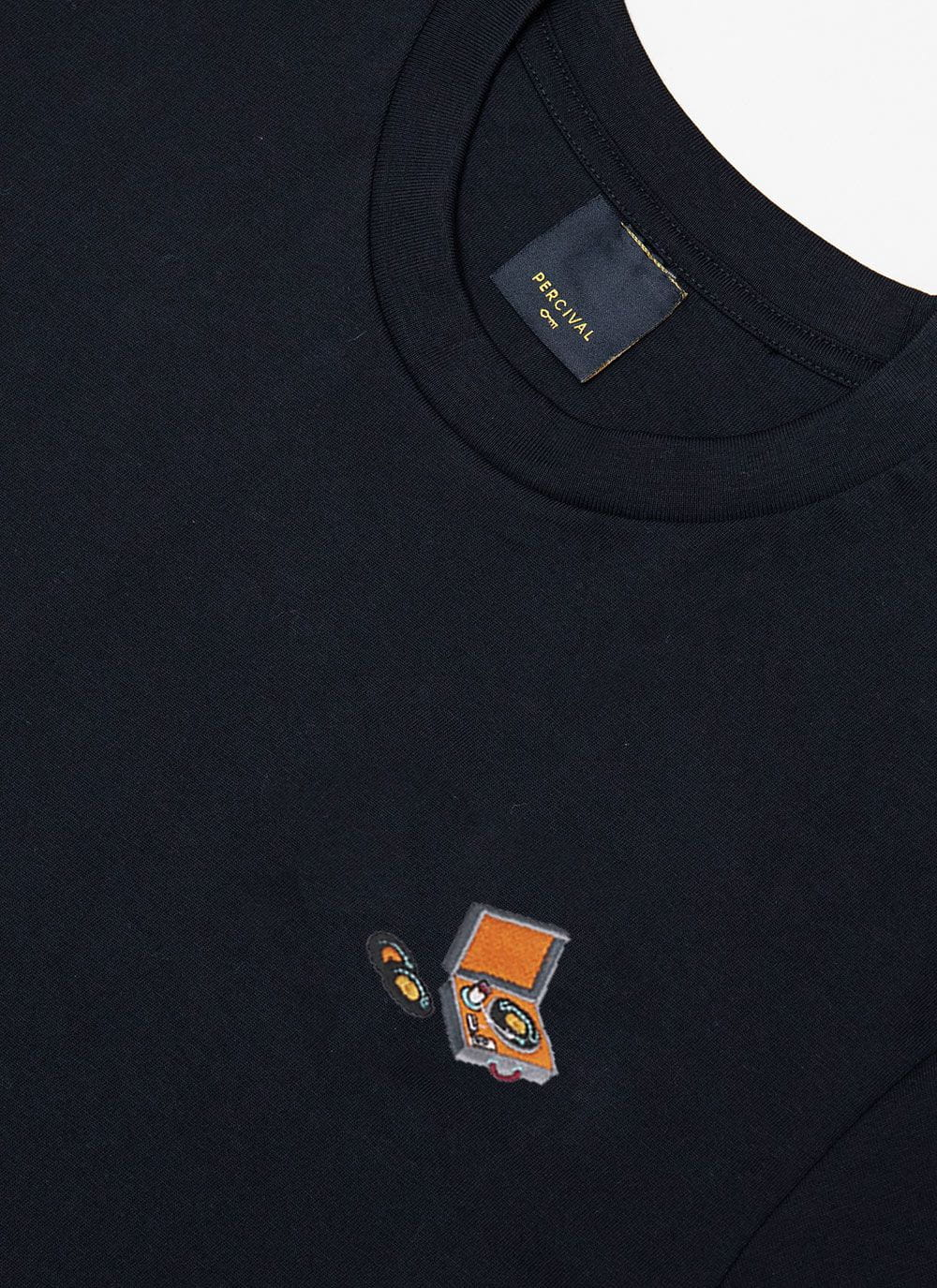• XS
• S
• M
• L
• XL
• XXL
• Select Size
Running Low• XS
• S
• M
• L
• XL
• XXL
• Select Size
Running Low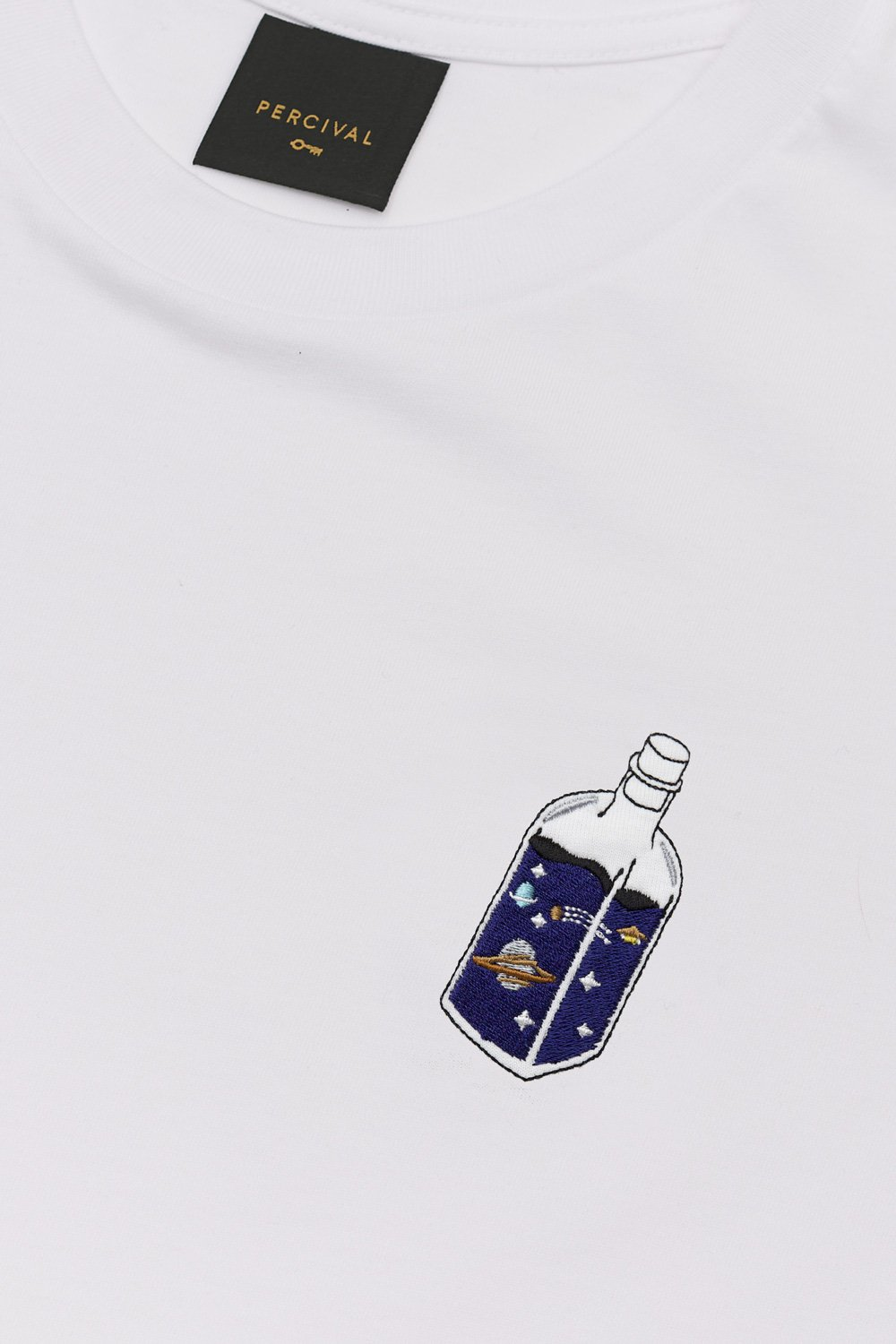• XS
• S
• M
• L
• XL
• XXL
• Select Size• XS
• S
• M
• L
• XL
• XXL
• Select Size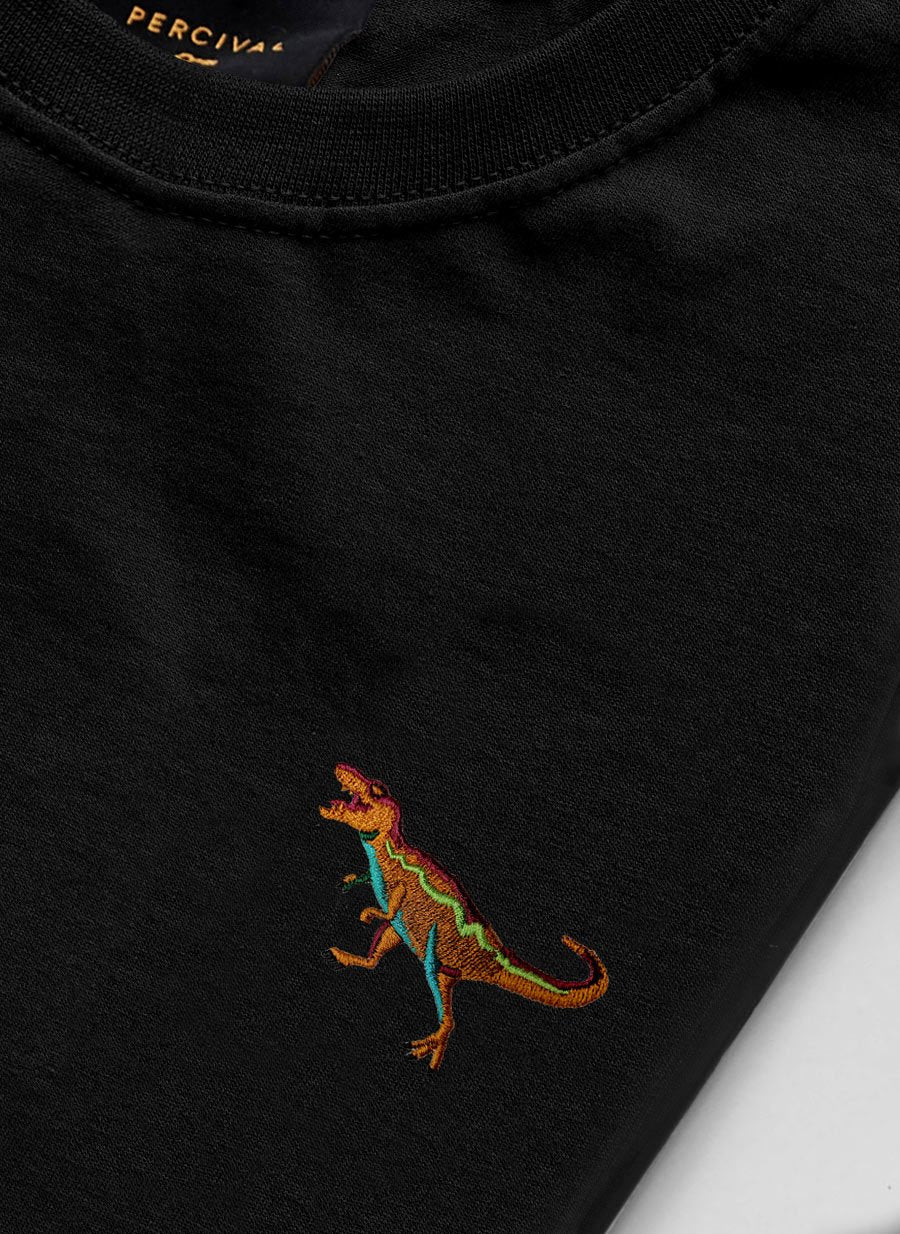• XS
• S
• M
• L
• XL
• XXL
• Select Size